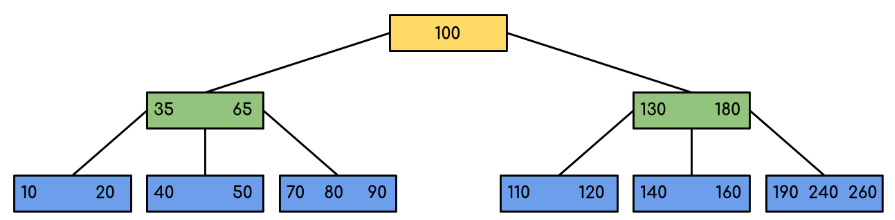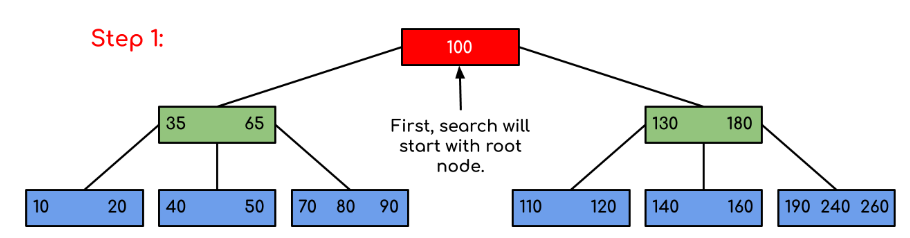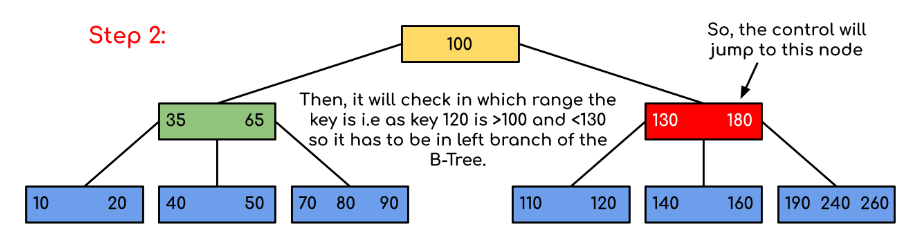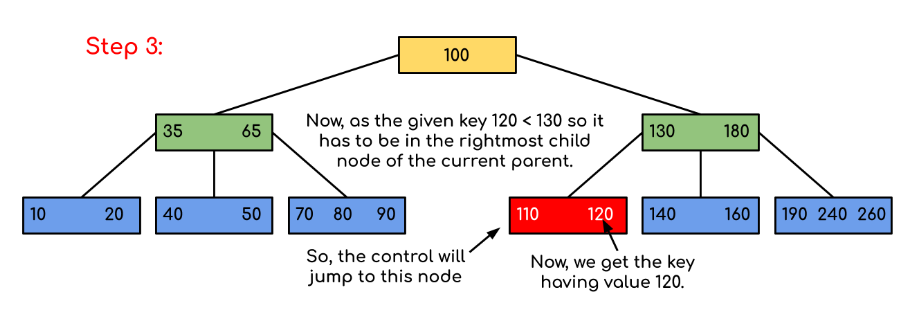GFG App
Open AppBrowser
Continue

# Introduction of B-Tree

The limitations of traditional binary search trees can be frustrating. Meet the B-Tree, the multi-talented data structure that can handle massive amounts of data with ease. When it comes to storing and searching large amounts of data, traditional binary search trees can become impractical due to their poor performance and high memory usage. B-Trees, also known as B-Tree or Balanced Tree, are a type of self-balancing tree that was specifically designed to overcome these limitations.

Unlike traditional binary search trees, B-Trees are characterized by the large number of keys that they can store in a single node, which is why they are also known as “large key” trees. Each node in a B-Tree can contain multiple keys, which allows the tree to have a larger branching factor and thus a shallower height. This shallow height leads to less disk I/O, which results in faster search and insertion operations. B-Trees are particularly well suited for storage systems that have slow, bulky data access such as hard drives, flash memory, and CD-ROMs.

B-Trees maintain balance by ensuring that each node has a minimum number of keys, so the tree is always balanced. This balance guarantees that the time complexity for operations such as insertion, deletion, and searching is always O(log n), regardless of the initial shape of the tree.

## Time Complexity of B-Tree:

Note: “n” is the total number of elements in the B-tree

## Properties of B-Tree:

• All leaves are at the same level.
• B-Tree is defined by the term minimum degree ‘t‘. The value of ‘t‘ depends upon disk block size.
• Every node except the root must contain at least t-1 keys. The root may contain a minimum of 1 key.
• All nodes (including root) may contain at most (2*t – 1) keys.
• Number of children of a node is equal to the number of keys in it plus 1.
• All keys of a node are sorted in increasing order. The child between two keys k1 and k2 contains all keys in the range from k1 and k2.
• B-Tree grows and shrinks from the root which is unlike Binary Search Tree. Binary Search Trees grow downward and also shrink from downward.
• Like other balanced Binary Search Trees, the time complexity to search, insert and delete is O(log n).
• Insertion of a Node in B-Tree happens only at Leaf Node.

Following is an example of a B-Tree of minimum order 5
Note: that in practical B-Trees, the value of the minimum order is much more than 5.We can see in the above diagram that all the leaf nodes are at the same level and all non-leafs have no empty sub-tree and have keys one less than the number of their children.

• The minimum height of the B-Tree that can exist with n number of nodes and m is the maximum number of children of a node can have is:• The maximum height of the B-Tree that can exist with n number of nodes and t is the minimum number of children that a non-root node can have is:and## Traversal in B-Tree:

Traversal is also similar to Inorder traversal of Binary Tree. We start from the leftmost child, recursively print the leftmost child, then repeat the same process for the remaining children and keys. In the end, recursively print the rightmost child.

## Search Operation in B-Tree:

Search is similar to the search in Binary Search Tree. Let the key to be searched is k.

• Start from the root and recursively traverse down.
• For every visited non-leaf node,
• If the node has the key, we simply return the node.
• Otherwise, we recur down to the appropriate child (The child which is just before the first greater key) of the node.
• If we reach a leaf node and don’t find k in the leaf node, then return NULL.

Searching a B-Tree is similar to searching a binary tree. The algorithm is similar and goes with recursion. At each level, the search is optimized as if the key value is not present in the range of the parent then the key is present in another branch. As these values limit the search they are also known as limiting values or separation values. If we reach a leaf node and don’t find the desired key then it will display NULL.

## C

 BtreeSearch(x, k)     i = 1           // n[x] means number of keys in x node     while i ≤ n[x] and k ≥ keyi[x]         do i = i + 1     if i  n[x] and k = keyi[x]         then return (x, i)        if leaf [x]         then return NIL     else         return BtreeSearch(ci[x], k)

## C++

 struct Node {     int n;     int key[MAX_KEYS];     Node* child[MAX_CHILDREN];     bool leaf; };   Node* BtreeSearch(Node* x, int k) {     int i = 0;     while (i < x->n && k >= x->key[i]) {         i++;     }     if (i < x->n && k == x->key[i]) {         return x;     }     if (x->leaf) {         return nullptr;     }     return BtreeSearch(x->child[i], k); }

## Java

 class Node {     int n;     int[] key = new int[MAX_KEYS];     Node[] child = new Node[MAX_CHILDREN];     boolean leaf; }   Node BtreeSearch(Node x, int k) {     int i = 0;     while (i < x.n && k >= x.key[i]) {         i++;     }     if (i < x.n && k == x.key[i]) {         return x;     }     if (x.leaf) {         return null;     }     return BtreeSearch(x.child[i], k); }

## Python3

 class Node:     def __init__(self):         self.n = 0         self.key =  * MAX_KEYS         self.child = [None] * MAX_CHILDREN         self.leaf = True   def BtreeSearch(x, k):     i = 0     while i < x.n and k >= x.key[i]:         i += 1     if i < x.n and k == x.key[i]:         return x     if x.leaf:         return None     return BtreeSearch(x.child[i], k)

## Javascript

 // Define a Node class with properties n, key, child, and leaf class Node {     constructor() {         this.n = 0;         this.key = new Array(MAX_KEYS);         this.child = new Array(MAX_CHILDREN);         this.leaf = false;     } }   // Define a function BtreeSearch that takes in a Node object x and an integer k function BtreeSearch(x, k) {     let i = 0;     while (i < x.n && k >= x.key[i]) {         i++;     }     if (i < x.n && k == x.key[i]) {         return x;     }     if (x.leaf) {         return null;     }     return BtreeSearch(x.child[i], k); }

## C#

 class Node {     public int n;     public int[] key = new int[MAX_KEYS];     public Node[] child = new Node[MAX_CHILDREN];     public bool leaf; }   Node BtreeSearch(Node x, int k) {     int i = 0;     while (i < x.n && k >= x.key[i]) {         i++;     }     if (i < x.n && k == x.key[i]) {         return x;     }     if (x.leaf) {         return null;     }     return BtreeSearch(x.child[i], k); }

Examples:

Input: Search 120 in the given B-Tree.Solution:In this example, we can see that our search was reduced by just limiting the chances where the key containing the value could be present. Similarly if within the above example we’ve to look for 180, then the control will stop at step 2 because the program will find that the key 180 is present within the current node. And similarly, if it’s to seek out 90 then as 90 < 100 so it’ll go to the left subtree automatically, and therefore the control flow will go similarly as shown within the above example.

Below is the implementation of the above approach:

## C++

 // C++ implementation of search() and traverse() methods #include  using namespace std;   // A BTree node class BTreeNode {     int* keys; // An array of keys     int t; // Minimum degree (defines the range for number            // of keys)     BTreeNode** C; // An array of child pointers     int n; // Current number of keys     bool leaf; // Is true when node is leaf. Otherwise false public:     BTreeNode(int _t, bool _leaf); // Constructor       // A function to traverse all nodes in a subtree rooted     // with this node     void traverse();       // A function to search a key in the subtree rooted with     // this node.     BTreeNode*     search(int k); // returns NULL if k is not present.       // Make the BTree friend of this so that we can access     // private members of this class in BTree functions     friend class BTree; };   // A BTree class BTree {     BTreeNode* root; // Pointer to root node     int t; // Minimum degree public:     // Constructor (Initializes tree as empty)     BTree(int _t)     {         root = NULL;         t = _t;     }       // function to traverse the tree     void traverse()     {         if (root != NULL)             root->traverse();     }       // function to search a key in this tree     BTreeNode* search(int k)     {         return (root == NULL) ? NULL : root->search(k);     } };   // Constructor for BTreeNode class BTreeNode::BTreeNode(int _t, bool _leaf) {     // Copy the given minimum degree and leaf property     t = _t;     leaf = _leaf;       // Allocate memory for maximum number of possible keys     // and child pointers     keys = new int[2 * t - 1];     C = new BTreeNode*[2 * t];       // Initialize the number of keys as 0     n = 0; }   // Function to traverse all nodes in a subtree rooted with // this node void BTreeNode::traverse() {     // There are n keys and n+1 children, traverse through n     // keys and first n children     int i;     for (i = 0; i < n; i++) {         // If this is not leaf, then before printing key[i],         // traverse the subtree rooted with child C[i].         if (leaf == false)             C[i]->traverse();         cout << " " << keys[i];     }       // Print the subtree rooted with last child     if (leaf == false)         C[i]->traverse(); }   // Function to search key k in subtree rooted with this node BTreeNode* BTreeNode::search(int k) {     // Find the first key greater than or equal to k     int i = 0;     while (i < n && k > keys[i])         i++;       // If the found key is equal to k, return this node     if (keys[i] == k)         return this;       // If the key is not found here and this is a leaf node     if (leaf == true)         return NULL;       // Go to the appropriate child     return C[i]->search(k); }

## Java

 // Java program to illustrate the sum of two numbers   // A BTree class Btree {     public BTreeNode root; // Pointer to root node     public int t; // Minimum degree       // Constructor (Initializes tree as empty)     Btree(int t)     {         this.root = null;         this.t = t;     }       // function to traverse the tree     public void traverse()     {         if (this.root != null)             this.root.traverse();         System.out.println();     }       // function to search a key in this tree     public BTreeNode search(int k)     {         if (this.root == null)             return null;         else             return this.root.search(k);     } }   // A BTree node class BTreeNode {     int[] keys; // An array of keys     int t; // Minimum degree (defines the range for number            // of keys)     BTreeNode[] C; // An array of child pointers     int n; // Current number of keys     boolean         leaf; // Is true when node is leaf. Otherwise false       // Constructor     BTreeNode(int t, boolean leaf)     {         this.t = t;         this.leaf = leaf;         this.keys = new int[2 * t - 1];         this.C = new BTreeNode[2 * t];         this.n = 0;     }       // A function to traverse all nodes in a subtree rooted     // with this node     public void traverse()     {           // There are n keys and n+1 children, traverse         // through n keys and first n children         int i = 0;         for (i = 0; i < this.n; i++) {               // If this is not leaf, then before printing             // key[i], traverse the subtree rooted with             // child C[i].             if (this.leaf == false) {                 C[i].traverse();             }             System.out.print(keys[i] + " ");         }           // Print the subtree rooted with last child         if (leaf == false)             C[i].traverse();     }       // A function to search a key in the subtree rooted with     // this node.     BTreeNode search(int k)     { // returns NULL if k is not present.           // Find the first key greater than or equal to k         int i = 0;         while (i < n && k > keys[i])             i++;           // If the found key is equal to k, return this node         if (keys[i] == k)             return this;           // If the key is not found here and this is a leaf         // node         if (leaf == true)             return null;           // Go to the appropriate child         return C[i].search(k);     } }

## Python3

 # Create a node class BTreeNode:   def __init__(self, leaf=False):     self.leaf = leaf     self.keys = []     self.child = []     # Tree class BTree:   def __init__(self, t):     self.root = BTreeNode(True)     self.t = t       # Insert node   def insert(self, k):     root = self.root     if len(root.keys) == (2 * self.t) - 1:       temp = BTreeNode()       self.root = temp       temp.child.insert(0, root)       self.split_child(temp, 0)       self.insert_non_full(temp, k)     else:       self.insert_non_full(root, k)       # Insert nonfull   def insert_non_full(self, x, k):     i = len(x.keys) - 1     if x.leaf:       x.keys.append((None, None))       while i >= 0 and k < x.keys[i]:         x.keys[i + 1] = x.keys[i]         i -= 1       x.keys[i + 1] = k     else:       while i >= 0 and k < x.keys[i]:         i -= 1       i += 1       if len(x.child[i].keys) == (2 * self.t) - 1:         self.split_child(x, i)         if k > x.keys[i]:           i += 1       self.insert_non_full(x.child[i], k)       # Split the child   def split_child(self, x, i):     t = self.t     y = x.child[i]     z = BTreeNode(y.leaf)     x.child.insert(i + 1, z)     x.keys.insert(i, y.keys[t - 1])     z.keys = y.keys[t: (2 * t) - 1]     y.keys = y.keys[0: t - 1]     if not y.leaf:       z.child = y.child[t: 2 * t]       y.child = y.child[0: t - 1]     # Print the tree   def print_tree(self, x, l=0):     print("Level ", l, " ", len(x.keys), end=":")     for i in x.keys:       print(i, end=" ")     print()     l += 1     if len(x.child) > 0:       for i in x.child:         self.print_tree(i, l)     # Search key in the tree   def search_key(self, k, x=None):     if x is not None:       i = 0       while i < len(x.keys) and k > x.keys[i]:         i += 1       if i < len(x.keys) and k == x.keys[i]:         return (x, i)       elif x.leaf:         return None       else:         return self.search_key(k, x.child[i])             else:       return self.search_key(k, self.root)     def main():   B = BTree(3)     for i in range(10):     B.insert((i, 2 * i))     B.print_tree(B.root)     if B.search_key(8) is not None:     print("\nFound")   else:     print("\nNot Found")     if __name__ == '__main__':   main()

## Javascript

 // Javascript program to illustrate the sum of two numbers   // A BTree class Btree {       // Constructor (Initializes tree as empty)     constructor(t)     {         this.root = null;         this.t = t;     }           // function to traverse the tree     traverse()     {         if (this.root != null)             this.root.traverse();         document.write("
");     }           // function to search a key in this tree     search(k)     {         if (this.root == null)             return null;         else             return this.root.search(k);     }       }   // A BTree node class BTreeNode {      // Constructor     constructor(t,leaf)     {         this.t = t;         this.leaf = leaf;         this.keys = new Array(2 * t - 1);         this.C = new Array(2 * t);         this.n = 0;     }     // A function to traverse all nodes in a subtree rooted with this node     traverse()     {         // There are n keys and n+1 children, traverse through n keys         // and first n children         let i = 0;         for (i = 0; i < this.n; i++) {                // If this is not leaf, then before printing key[i],             // traverse the subtree rooted with child C[i].             if (this.leaf == false) {                 C[i].traverse();             }             document.write(keys[i] + " ");         }            // Print the subtree rooted with last child         if (leaf == false)             C[i].traverse();     }           // A function to search a key in the subtree rooted with this node.     search(k)    // returns NULL if k is not present.     {               // Find the first key greater than or equal to k         let i = 0;         while (i < n && k > keys[i])             i++;            // If the found key is equal to k, return this node         if (keys[i] == k)             return this;            // If the key is not found here and this is a leaf node         if (leaf == true)             return null;            // Go to the appropriate child         return C[i].search(k);     } }   // This code is contributed by patel2127

## C#

 // C# program to illustrate the sum of two numbers using System;   // A BTree class Btree {     public BTreeNode root; // Pointer to root node     public int t; // Minimum degree       // Constructor (Initializes tree as empty)     Btree(int t)     {         this.root = null;         this.t = t;     }       // function to traverse the tree     public void traverse()     {         if (this.root != null)             this.root.traverse();         Console.WriteLine();     }       // function to search a key in this tree     public BTreeNode search(int k)     {         if (this.root == null)             return null;         else             return this.root.search(k);     } }   // A BTree node class BTreeNode {     int[] keys; // An array of keys     int t; // Minimum degree (defines the range for number            // of keys)     BTreeNode[] C; // An array of child pointers     int n; // Current number of keys     bool leaf; // Is true when node is leaf. Otherwise false       // Constructor     BTreeNode(int t, bool leaf)     {         this.t = t;         this.leaf = leaf;         this.keys = new int[2 * t - 1];         this.C = new BTreeNode[2 * t];         this.n = 0;     }       // A function to traverse all nodes in a subtree rooted     // with this node     public void traverse()     {           // There are n keys and n+1 children, traverse         // through n keys and first n children         int i = 0;         for (i = 0; i < this.n; i++) {               // If this is not leaf, then before printing             // key[i], traverse the subtree rooted with             // child C[i].             if (this.leaf == false) {                 C[i].traverse();             }             Console.Write(keys[i] + " ");         }           // Print the subtree rooted with last child         if (leaf == false)             C[i].traverse();     }       // A function to search a key in the subtree rooted with     // this node.     public BTreeNode search(int k)     { // returns NULL if k is not present.           // Find the first key greater than or equal to k         int i = 0;         while (i < n && k > keys[i])             i++;           // If the found key is equal to k, return this node         if (keys[i] == k)             return this;           // If the key is not found here and this is a leaf         // node         if (leaf == true)             return null;           // Go to the appropriate child         return C[i].search(k);     } }   // This code is contributed by Rajput-Ji

Note: The above code doesn’t contain the driver program. We will be covering the complete program in our next post on B-Tree Insertion.

There are two conventions to define a B-Tree, one is to define by minimum degree, second is to define by order. We have followed the minimum degree convention and will be following the same in coming posts on B-Tree. The variable names used in the above program are also kept the same

## Applications of B-Trees:

• It is used in large databases to access data stored on the disk
• Searching for data in a data set can be achieved in significantly less time using the B-Tree
• With the indexing feature, multilevel indexing can be achieved.
• Most of the servers also use the B-tree approach.
• B-Trees are used in CAD systems to organize and search geometric data.
• B-Trees are also used in other areas such as natural language processing, computer networks, and cryptography.

•  B-Trees have a guaranteed time complexity of O(log n) for basic operations like insertion, deletion, and searching, which makes them suitable for large data sets and real-time applications.
•  B-Trees are self-balancing.
• High-concurrency and high-throughput.
• Efficient storage utilization.

• B-Trees are based on disk-based data structures and can have a high disk usage.
• Not the best for all cases.
• Slow in comparison to other data structures.

Insertion and Deletion:
B-Tree Insertion
B-Tree Deletion